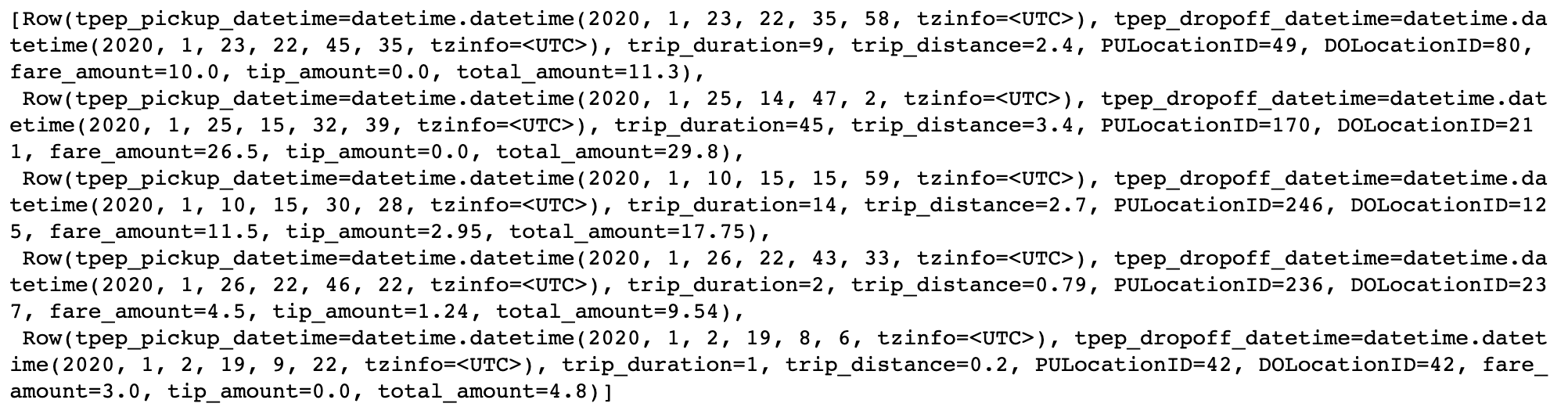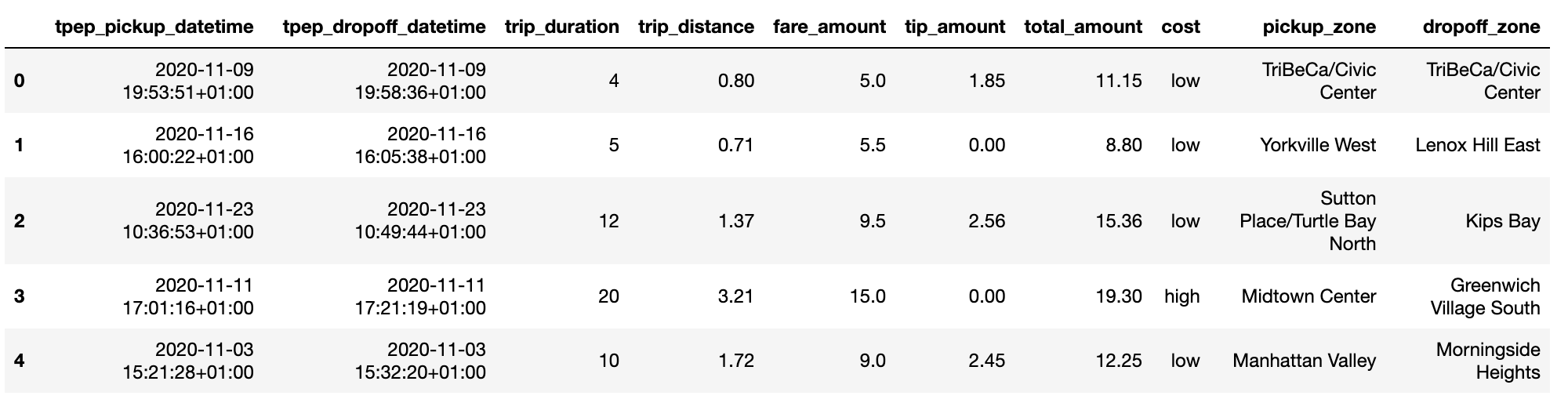# Using Snowpark Python in Dataiku: basics#

Pre-requisites

## What is Snowpark?#

Snowpark is a set of libraries to programmatically access and process data in Snowflake using languages like Python, Java or Scala. It allows the user to manipulate DataFrames similarly to Pandas or PySpark. The Snowflake documentation provides more details on how Snowpark works under the hood.

In this tutorial, you will work with the NYC_trips and NYC_zones Datasets to discover a few features of the Snowpark Python API and how they can be used within Dataiku to:

• Faciliate reading and writing Snowflake Datasets.

• Perform useful/common data transformation.

• Leverage User Defined Functions (UDFs).

## Creating a Session#

Whether using Snowpark Python in a Python recipe or notebook, you’ll first need to create a Snowpark Session.

A Session object is used to establish a connection with a Snowflake database. Normally, this Session would need to be instantiated with the user manually providing credentials such as the user id and password. However, the get_session() method reads all the necessary parameters from the Snowflake connection in DSS and thus exempts the user from having to handle credentials manually.

Start by creating a Jupyter notebook with the code environment mentioned in the pre-requisites and instantiate your Session object:

from dataiku.snowpark import DkuSnowpark

sp = DkuSnowpark()
# Replace with the name of your Snowflake connection
session = sp.get_session(connection_name="YOUR-CONNECTION-NAME")


Before working with the data, you first need to read it, more precisely to load it from a Snowflake table into a Snowpark Python DataFrame. With your session variable, create a Snowpark DataFrame using one of the following ways:

### Option 1: with the Dataiku API#

The easiest way to query a Snowpark DataFrame is by using the get_dataframe() method and passing a dataiku.Dataset object. The get_dataframe() can optionally be given a Snowpark Session argument. Dataiku will use the session created above or create a new one if no argument is passed.

import dataiku
NYC_trips = dataiku.Dataset("NYC_trips")
df_trips = sp.get_dataframe(dataset=NYC_trips)


### Option 2: with a SQL query#

Using the session object, a DataFrame can be created from a SQL query.

# Get the name of the dataiku.Dataset's underlying Snowflake table.
trips_table_name = NYC_trips.get_location_info().get('info', {}).get('table')
df_trips = session.sql(f"Select * from {trips_table_name}")


Unlike Pandas DataFrames, Snowpark Python DataFrames are lazily evaluated. This means that they, and any subsequent operation applied to them, are not immediately executed.

Instead, they are recorded in a Directed Acyclic Graph (DAG) that is evaluated only upon the calling of certain methods (collect(), take(), show(), toPandas()).

This lazy evaluation minimizes traffic between the Snowflake warehouse and the client as well as client-side memory usage.

## Retrieving rows#

• The take(n) method is the only method that allows users to pull and check n rows from the Snowpark DataFrame. Yet, it is arguably not the most pleasant way of checking a DataFrame’s content.

# Retrieve 5 rows
df_trips.take(5)• The toPandas() method converts the Snowpark DataFrame into a more aesthetically-pleasing Pandas DataFrame. Avoid using this method if the data is too large to fit in memory. Instead, leverage the to_pandas_batches() method. Alternatively, you can use a limit statement before retrieving the results as a Pandas DataFrame.

df_trips.limit(5).toPandas()## Common operations#

The following paragraphs illustrate a few examples of basic data manipulation using DataFrames:

### Selecting column(s)#

Snowflake stores unquoted column names in uppercase. Be sure to use double quotes for case-sensitive column names. Using the select method returns a DataFrame:

from snowflake.snowpark.functions import col

fare_amount = df_trips.select([col('"fare_amount"'),col('"tip_amount"')])

# Shorter equivalent version:
fare_amount = df_trips.select(['"fare_amount"','"tip_amount"'])


### Computing the average of a column#

Collect the mean fare_amount. This returns a 1-element list of type snowflake.snowpark.row.Row:

from snowflake.snowpark.functions import mean

avg_row = df_trips.select(mean(col('"fare_amount"'))).collect()
avg_row # results [Row(AVG("FARE_AMOUNT")=12.556332926005984)]


You can access the value as follows:

avg = avg_row.asDict().get('AVG("FARE_AMOUNT")')


### Creating a new column from a case expression#

Leverage the withColumn() method to create a new column indicating whether a trip’s fare was above average. That new column is the result of a case expression (when() and otherwise()):

from snowflake.snowpark.functions import when

df_trips = df_trips.withColumn('"cost"', when(col('"fare_amount"') > avg, "high")\
.otherwise("low"))

# Check the first five rows
df_trips.select(['"cost"', '"fare_amount"']).take(5)### Joining two tables#

The NYC_trips contains a pick up and drop off location id (PULocationID and DOLocationID). We can map those location ids to their corresponding zone names using the NYC_zones Dataset.

To do so, perform two consecutive joins on the OBJECTID column in the NYC zone Dataset.

import pandas as pd

# Get the NYC_zones Dataset object
NYC_zones = dataiku.Dataset("NYC_zones")
df_zones = sp.get_dataframe(NYC_zones)

df_zones.toPandas()Finally, perform the two consecutive left joins. Note how you are able to chain different operations including withColumnRenamed() to rename the zone column and drop() to remove other columns from the NYC_zones Dataset:

df = df_trips.join(df_zones, col('"PULocationID"')==col('"OBJECTID"'))\
.withColumnRenamed(col('"zone"'), '"pickup_zone"')\
.drop([col('"OBJECTID"'), col('"PULocationID"'), col('"borough"')])\
.join(df_zones, col('"DOLocationID"')==col('"OBJECTID"'))\
.withColumnRenamed(col('"zone"'), '"dropoff_zone"')\
.drop([col('"OBJECTID"'), col('"DOLocationID"'),col('"borough"')])


### Group By#

Count the number of trips by pickup zone among expensive trips. Use the filter() method to remove cheaper trips. Then use the groupBy() method to group by pickup_zone, count() the number of trips and sort() them by descending order. Finally, call the toPandas() method to store the results of the group by as a Pandas DataFrame.

results_count_df = df.filter((col('"cost"')=="low"))\
.groupBy(col('"pickup_zone"'))\
.count()\
.sort(col('"COUNT"'), ascending=False)\
.toPandas()

results_count_df## User Defined Functions (UDF)#

Snowpark’s use would rather be limited if it wasn’t for UDFs.

A User Defined Functions (UDF) is a function that, for a single row, takes the values of one or several cells from that row, and returns a new value.

UDFs effectively allow users to transform data using custom complex logic beyond what’s possible in pure SQL. This includes the use of any Python packages.

To be used, UDFs first need to be registered so that at execution time they can be properly sent to the Snowflake servers. In this section, you will see a simple UDF example and how to register it.

### Registering a UDF#

• The first option to register a UDF is to use either the register() or the udf() function. In the following code block is a simple UDF example that computes the tip percentage over the taxi ride total fare amount:

from snowflake.snowpark.functions import udf
from snowflake.snowpark.types import FloatType

def get_tip_pct(tip_amount, fare_amount):
return tip_amount/fare_amount

# Register with register()
get_tip_pct_udf = session.udf.register(get_tip_pct, input_types=[FloatType(), FloatType()],
return_type=FloatType())

# Register with udf()
get_tip_pct_udf = udf(get_tip_pct, input_types=[FloatType(), FloatType()],
return_type=FloatType())


• An alternative way of registering the get_tip_pct() function as a UDF is to decorate your function with @udf . If you choose this way, you will need to specify the input and output types directly in the Python function.

@udf
def get_tip_pct(tip_amount:float, fare_amount:float) -> float:
return tip_amount/fare_amount



### Applying a UDF#

Now that the UDF is registered, you can use it to generate new columns in your DataFrame using withColumn():

df = df.withColumn('"tip_pct"', get_tip_pct_udf('"tip_amount"', '"fare_amount"' ))


After running this code, you should be able to see that the tip_pct column was created in the df DataFrame.

## Writing a DataFrame into a Snowflake Dataset#

In a Python recipe, you will likely want to write Snowpark DataFrame into a Snowflake output Dataset. We recommend using the write_with_schema() method of the DkuSnowpark class. This method runs the saveAsTable() Snowpark Python method to save the contents of a DataFrame into a Snowflake table.

ouput_dataset = dataiku.Dataset("my_output_dataset")
sp.write_with_schema(ouput_dataset, df)


Warning

You should avoid converting a Snowpark Python DataFrame to a Pandas DataFrame before writing the output Dataset. In the following example, using the toPandas() method will create the Pandas DataFrame locally, further increasing memory usage and potentially leading to resource shortage issues.

ouput_dataset = dataiku.Dataset("my_output_dataset")
# Load the ENTIRE DataFrame in memory (NOT optimal !!)
ouput_dataset.write_with_schema(df.toPandas())


## Wrapping up#

Congratulations, you now know how to work with Snowpark Python within Dataiku! To go further, here are some useful links: